## de la Matière Condensée

Postdoctoral researcher at LPTMC, Sorbonne Université

e-mail: aurelien (dot) grabsch (at) sorbonne-universite.fr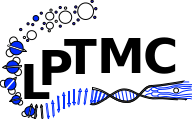### Research

Keywords: statistical physics, condensed matter theory, random matrix theory, diffusion in crowded environments, coherent quantum transport, topology in condensed matter, Majorana zero modes, disordered systems, large deviations, stochastic processes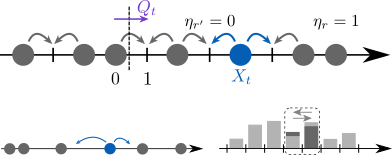I am interested in the physics of single-file systems. These are systems in which particles cannot bypass each other. Following the dynamics of a tracer particle, we observe a subdiffusive behaviour, which originates from strong bath-tracer correlations. Focusing on paradigmatic models of single-file diffusion, such as the Simple Exclusion Process (SEP), I use analytical tools (master equations, large deviations, ...) to characterise the bath-tracer correlations. In turn, the knowledge of these correlations allow to fully characterise the dynamics of the tracer particle.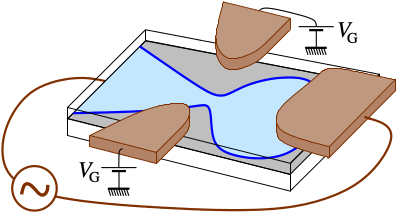I am interested in the applications of random matrix theory (RMT) to quantum transport. The complex dynamics of chaotic cavities, like quantum dots, can be well described by a statistical approach. This consists in taking a random scattering matrix to characterize transport through the system. From this matrix can be constructed the Wigner-Smith time-delay matrix, which was shown to belong to the (inverse) Laguerre ensemble of RMT. Many relevant physical quantities (like conductance, resistance,...) take the form of linear statistics of the eigenvalues of this matrix (the proper time delays).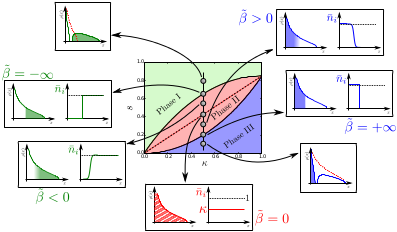The distribution of these linear statistics is obtained by a Coulomb gas method. This consists in interpreting the eigenvalues of a random matrix as the positions of particles in a 1D gas, with logarithmic repulsion. Determining the distribution of the linear statistics reduces to finding the optimal configuration of the gas under a constraint. An interesting feature is the possibility of phase transitions driven by the constraint.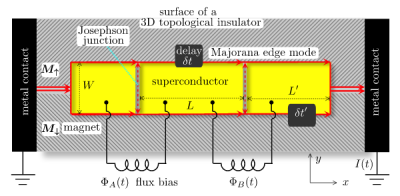Topological superconductors can support Majorana zero-modes (midgap states bound to a defect). These zero modes have non-Abelian statistics: they are neither bosons nor fermions, and can be used for the realisation of topologically protected quantum computations. Topological superconductors also possess non-Abelian excitations of the chiral edge modes: the edge vortices. Unlike the Majorana zero-modes which are fixed, the edge vortices have the advantage of propagating along the chiral edge modes. I am investigating the possibility to demonstrate the non-Abelian nature of the edge vortices, and their possible use for the realisation of topologically protected quantum computations.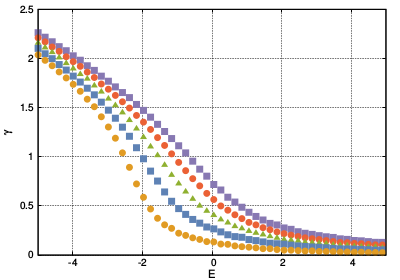I am also interested in disordered systems. It is well known that wave functions in 1D in a random potential are localized (Anderson localization). The localization length have been computed for diverse models of disorder. However the 2D case is still out of reach. I mostly focus on models of multichannel disordered wires which describe an intermediate situation, using matrix Langevin equations.

### Teaching

Enseignements à l'Université Paris-Sud :

• Cours-TD de physique quantique (2015-2018)
• TD Programmation et données numériques (2015-2017)